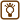## Unknown Compounds

### Task number: 1272

Two elements X a Y form two compounds XY and XY2.

Find these two elements knowing that one kilogram of the first compound corresponds to the amount of substance of 35.71 mol, and one kilogram of the second compound corresponds to the amount of substance of 22.73 mol.

• #### Hint

Based on the given data, first determine the molar mass of both compounds.

Then, using the system of two equations calculate the molar mass of the two unknown elements. Knowing the molar masses of the elements, you can find these elements in the periodic table.

• #### Analysis

Knowing what amount of substance (= „how many moles“) corresponds to one kilogram of each substance we can calculate their molar masses. Using the familiar molecular formula we can prepare two equations with two unknown variables – the molar masses of the two elements X and Y we are looking for. We solve the equation system and by the obtained molar masses of the elements we can find these elements in the periodic table.

• #### Solution

First we determine the molar mass of both compounds:

$M_{1}\,=\,\frac{m}{n_1}\,=\,\frac{1\,\mathrm{kg}}{35.71\,\mathrm{mol}}\,=\,0.028 \,\mathrm{kg\,mol}^{-1}$ $M_{1}\,=\,28\,\mathrm{g\,mol}^{-1} \hspace{20px} \Rightarrow \hspace{20px} M_r\mathrm{(XY)}\,=\,28$

$M_{2}\,=\,\frac{m}{n_2}\,=\,\frac{1\,\mathrm{kg}}{22.73\,\mathrm{mol}}\,=\,0.044 \,\mathrm{kg\,mol}^{-1}$ $M_{2}\,=\,44\,\mathrm{g\,mol}^{-1} \hspace{20px} \Rightarrow \hspace{20px} M_r\mathrm{(XY_2)}\,=\,44$

We know that molar mass with the unit g mol-1 is numerically equal to the dimensionless relative molecular mass. Thus we can write the equations to calculate the relative molecular mass of both compounds using the relative atomic mass of elements X and Y.

$M_r\mathrm{(XY)} \,=\,A_r\mathrm{(X)} \,+\, A_r\mathrm{(Y)}$ $M_r\mathrm{(XY_2)}\,=\, A_r\mathrm{(X)} \,+\, 2 A_r\mathrm{(Y)}$

Now we substitute the set values and unknown relative atomic mass of both elements for clarity denote as x and y:

$x \,+\, y \,=\, 28$ $x \,+\, 2y \,=\, 44$

This is a simple system of two equations in two variables. Subtracting the first equation from the second one we get:

$(x \,+\, 2y) - (x \,+ \,y) \,=\, 44\,-\, 28$ $y \,=\, 16$

and from the first equation we get:

$x \,=\, 28 \,-\, y \,=\, 28 \,-\, 16 \,=\, 12$

The relative atomic mass 12 belongs to carbon and the relative atomic mass 16 belongs to oxygen.

• #### Answer

The unknown elements are carbon and oxygen and the compounds are carbon monoxide CO and carbon dioxide CO2.